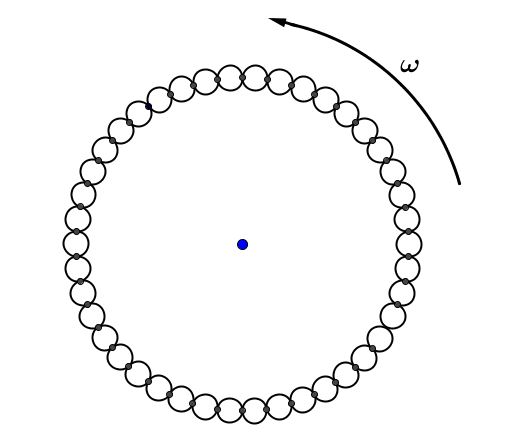# Waves waves waves!A circular loop of rope of length L rotates with uniform angular velocity $\omega$ about an axis through its center on a horizontal smooth platform. A small displacement is given to radially which makes it produce the pulses. Find the velocity of the pulse (in $\text{m/s}$) with respect to the rope.

Details and Assumptions

• The length of the rope is $L = 3 \text{ m}$.

• The angular velocity, $\omega = 20 \pi \text{ rad/s}$.

×